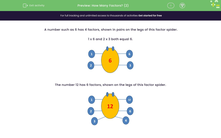# Count the Factors

In this worksheet, students will state how many factors a number has by looking at its 'factor spider'!Key stage:  KS 2

Curriculum topic:   Number: Multiplication and Division

Curriculum subtopic:   Identify Multiples and Factors

Popular topics:   Factors worksheets

Difficulty level:#### Worksheet Overview

In this activity, we are going to be looking at the factors of numbers.

The number 6 has four factors, shown in pairs on the legs of this factor spider.

The factor pairs of 6 are:

1 and 6

2 and 3

So, it has two factor pairs and four factors: 1, 2, 3 and 6The number 12 has six factors, shown on the legs of this factor spider.The factor pairs of 12 are:
1 and 12
2 and 6
3 and 4

So, there are three factor pairs and six factors: 1, 2, 3, 4, 6 and 12

Look at the factor spider for the number 9, which has three factors.

It has two factor pairs:

1 and 9

3 and 3

But only three factors: 1, 3 and 9

Note that the factor 3 should only be counted once.Let's try some questions now.

### What is EdPlace?

We're your National Curriculum aligned online education content provider helping each child succeed in English, maths and science from year 1 to GCSE. With an EdPlace account you’ll be able to track and measure progress, helping each child achieve their best. We build confidence and attainment by personalising each child’s learning at a level that suits them.

Get started